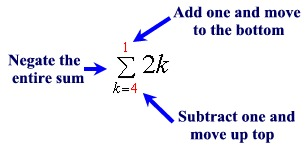Evaluating Summation Notation

A shorthand notation is used to represent sums with more than a few terms.  This shorthand notation uses the Greek letter sigma (which denotes sum).  If we have a rule such as f (n) which assigns the value f (n) to each integer n in the set of integers {j, j + 1, j + 2, ... k}, we can represent the sum as: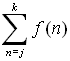=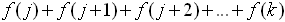On the Calculator:
 Example 1: Evaluate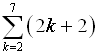Use the template to bring up the summation notation (sigma). Pressand choose the sigma notation. ENTER.Use the TAB key to move through the template entering your values.Example 2: Evaluate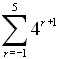Notice that the starting value is negative.Investigate! What happens when the top value is one less than the bottom value? Evaluate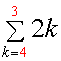If the top value is one less than the bottom value, the answer is always 0.What happens when the top value is more than one less than the bottom value? Evaluate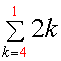This equals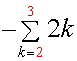To get this new equivalent summation, add one to the top value and move it to the bottom. Subtract one from the bottom value and move it to the top. Negate this entire new sum.